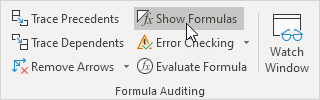# show formulas shortcut key in excel

Feb 06, 2020 • edited Feb 07, 2020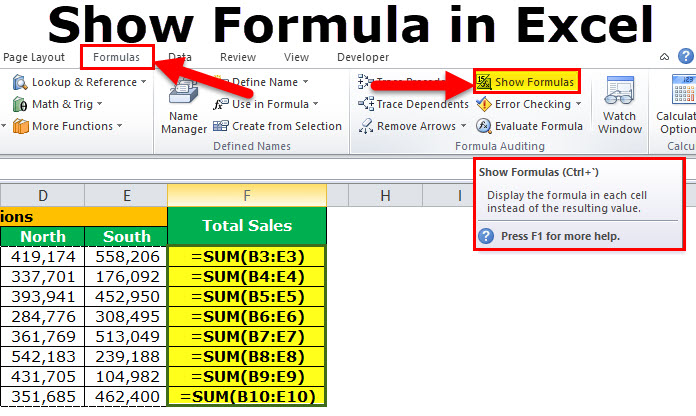# Show Formulas

Excel provides a really simple and quick way to show formulas in cells, and in a moment, you will make sure of this.

By default, Excel shows the results of formulas. To show the formulas instead of their results, press CTRL + ` (you can find this key above the tab key).

1. When you select a cell, Excel shows the formula of the cell in the formula bar.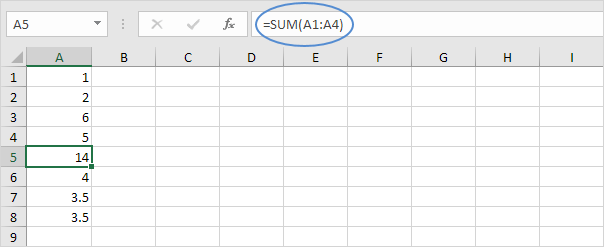2. To display all formulas, in all cells, press CTRL + ` (you can find this key above the tab key).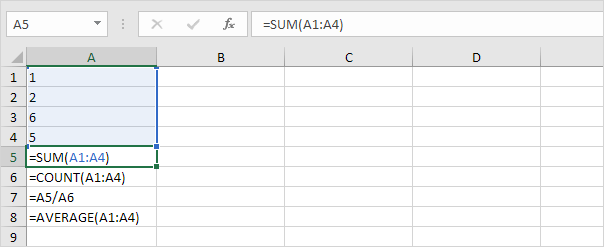3. Press ↓ twice.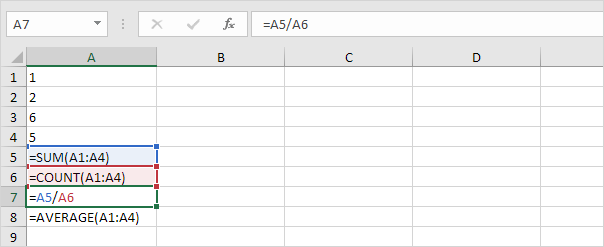Note: as you can see, Excel highlights all cells that are referenced by a formula.

4. To hide all formulas, press CTRL + ` again.

5. If you can't find the grave accent (`) on your keyboard, on the Formulas tab, in the Formula Auditing group, click Show Formulas.